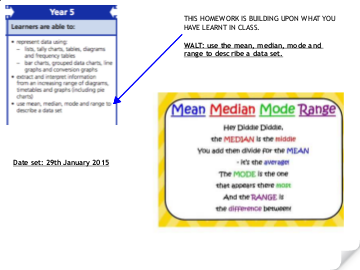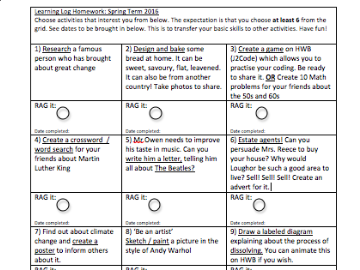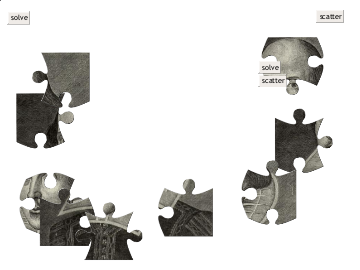# Monthly Archives: January 2016

## TestView post

## dissolvingView post

## dissolvingView post

## mean median range modeView post

## Year 5 spellings ‘cian’ words 25th January 2016

You have been working on understanding ‘cian’ words in class. You will have a homework sheet, which will ask you to alphabetically order words. Once this is done, Mrs Reece wants you to learn the spellings. The words you have are from the list below:

dietician
electrician
magician
musician
optician
physician
technician
mathematician
politician
beautician

LEARN THE WORDS FOR A SPELLING CHALLENGE ON FRIDAY! Have fun. Think of all of the fun ways in which you can learn the words.

## Maths Homework 23/1 /16

In class, we have been practising the inverse when checking answers. Please try to have a go at some of these at home.

e.g.: 234 – 121 =

Estimate first, by rounding to the nearest 10: 230 – 120 =

230- 100 – 20 = 110

Now do it:

2 3 4
– 1 2 1
1 1 3

Then CHECK it:Use the opposite symbol to the one used in the sum, which would be a

1 1 3
1 2 1
2 3 4                   CORRECT!

Have a go at these:

1) 524 – 231 =

2) 332 – 112 =

3) 987 – 206 =

4) 901 – 432 =

5) 443 + 221 =

6) 201 + 109 =

## Maths Homework

We explored fractional quantities last term and have also revised them in class. Your task is to complete the fractional quantity sheet in your books. Please do not write on the sheets, but copy them into your books neatly.

To find fractional quantities of a number:

e.g.

4/5 of 20.

1) First ask yourself, ‘How many fifths? 5. So how many 5s are in 20?’ What does this mean? Well it means, divide the number by 5.

1/5 = 20 ÷ 5  = 4

But I need 4 of them! So that would be 4+4+4+4= 16 (or 4 x 4=16)

So 4/5 of 20 is 16.

Mrs R

## ‘ou’ words

young
touch
double
trouble
country
enough
couple
cousin
rough
tough
southern
nourish
courage
ambitious
anxious
boisterous

Extend:

As well as learning these spellings thoroughly, can you think of some interesting sentences to put them into.

To be challenged 15/1/16

## Spring Term Log HomeworkView post